NCERT Exemplar - MCQs

Chapter 6 Class 12 Application of Derivatives
Serial order wise

## (C) 5x – y = 2   (D) 5x + y = 2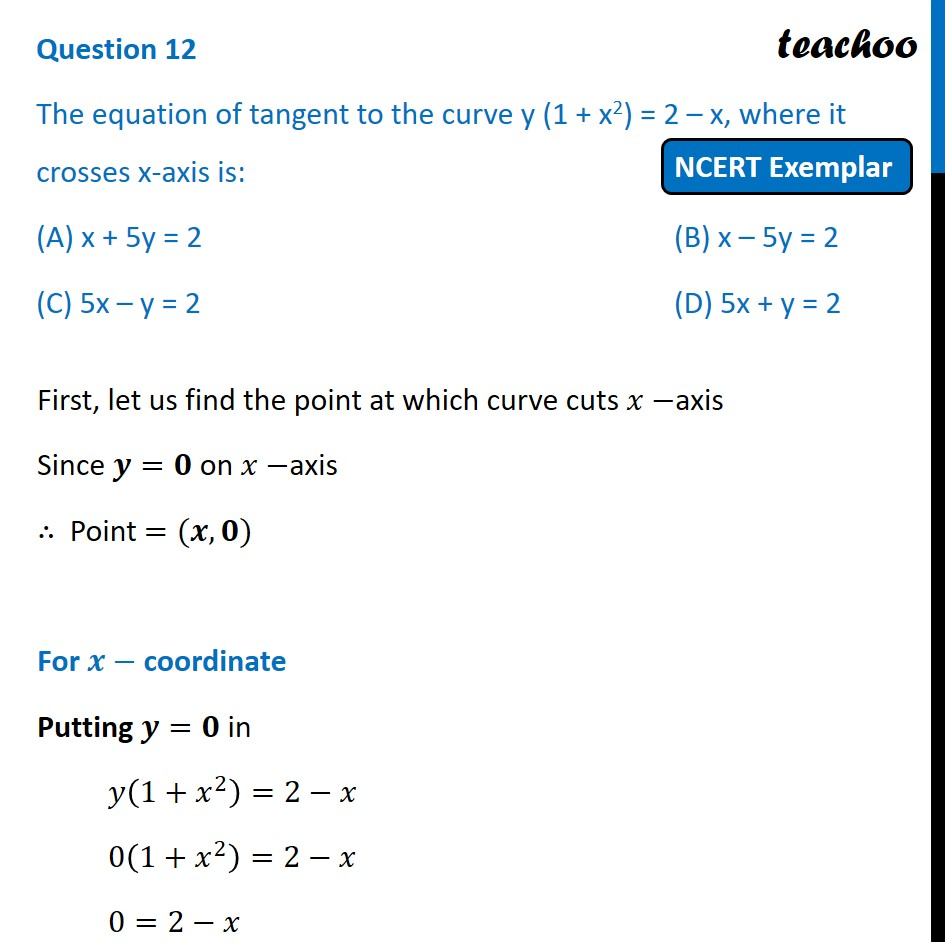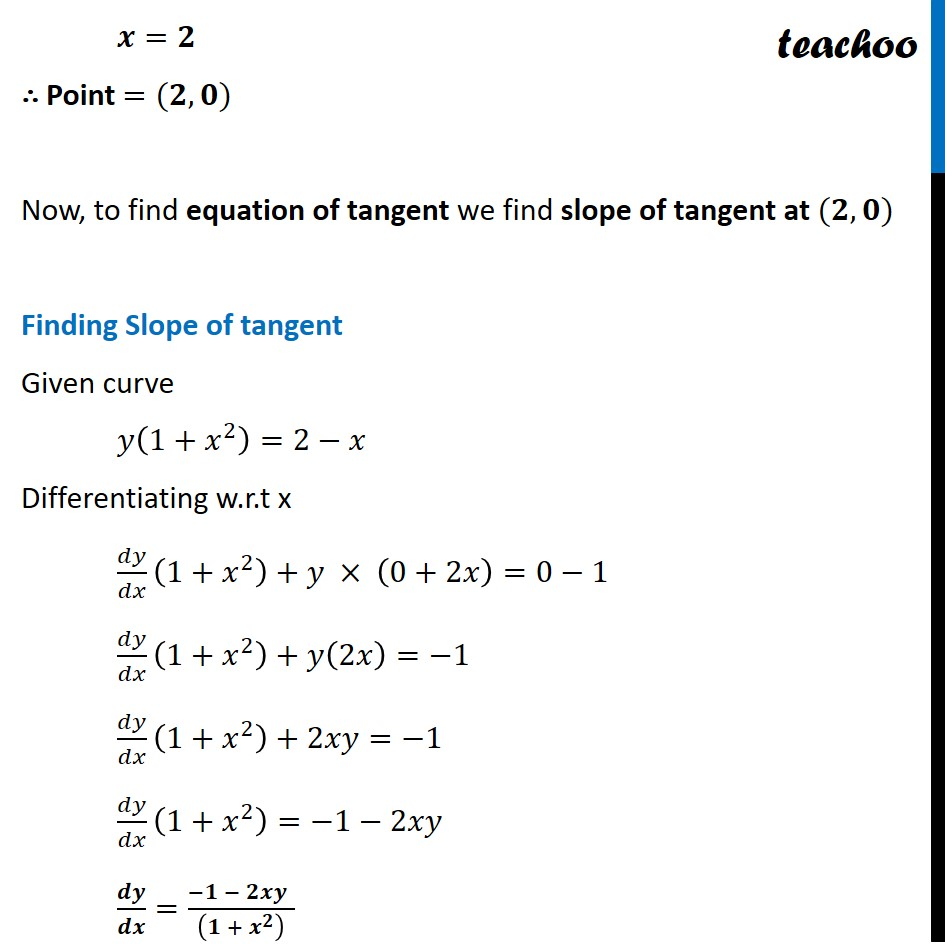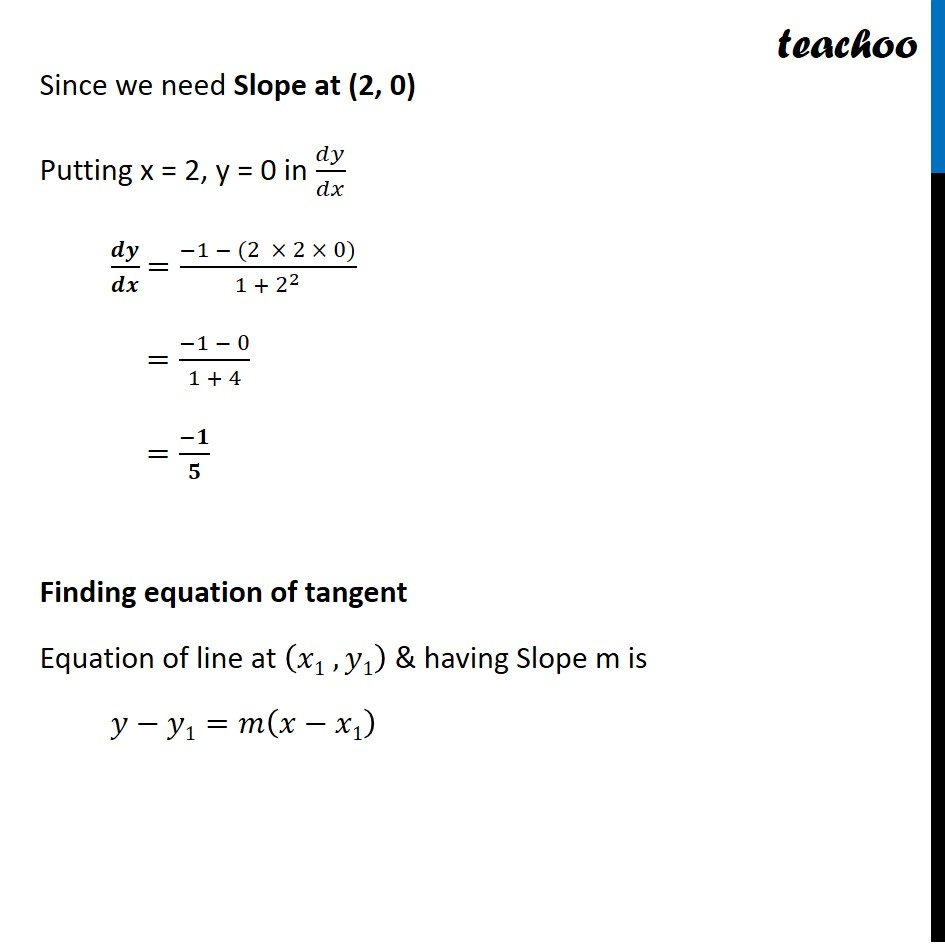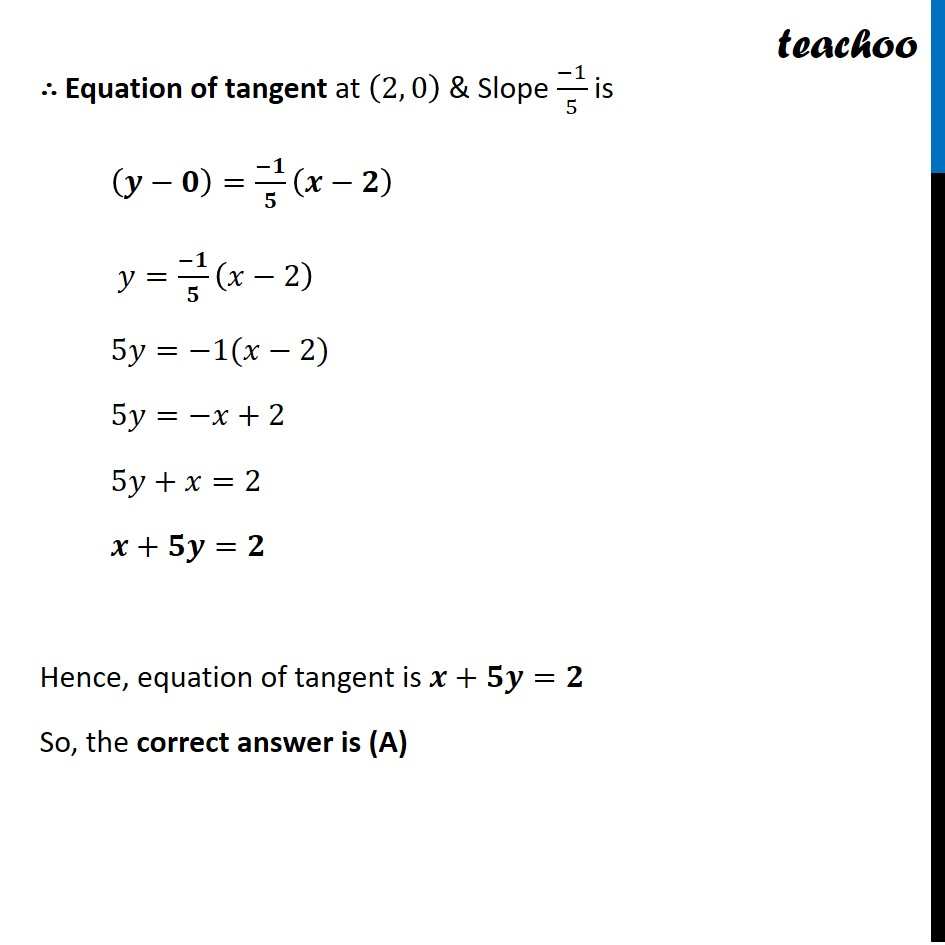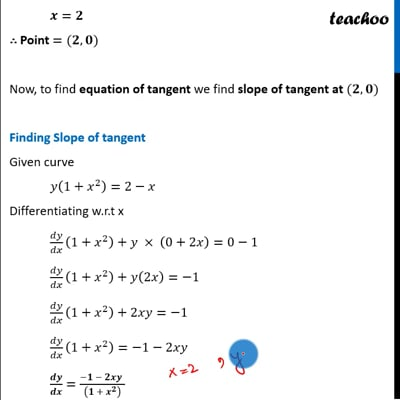This video is only available for Teachoo black users

Learn in your speed, with individual attention - Teachoo Maths 1-on-1 Class

### Transcript

Question 10 The equation of tangent to the curve y (1 + x2) = 2 – x, where it crosses x-axis is: (A) x + 5y = 2 (B) x – 5y = 2 (C) 5x – y = 2 (D) 5x + y = 2 First, let us find the point at which curve cuts 𝑥−axis Since 𝒚=𝟎 on 𝑥−axis ∴ Point =(𝒙,𝟎) For 𝒙− coordinate Putting 𝒚=𝟎 in 𝑦(1+𝑥^2 )=2−𝑥 0(1+𝑥^2 )=2−𝑥 0=2−𝑥 𝒙=𝟐 ∴ Point =(𝟐,𝟎) Now, to find equation of tangent we find slope of tangent at (𝟐, 𝟎) Finding Slope of tangent Given curve 𝑦(1+𝑥^2 )=2−𝑥 Differentiating w.r.t x 𝑑𝑦/𝑑𝑥 (1+𝑥^2 )+𝑦 × (0+2𝑥)=0−1 𝑑𝑦/𝑑𝑥 (1+𝑥^2 )+𝑦(2𝑥)=−1 𝑑𝑦/𝑑𝑥 (1+𝑥^2 )+2𝑥𝑦=−1 𝑑𝑦/𝑑𝑥 (1+𝑥^2 )=−1−2𝑥𝑦 𝒅𝒚/𝒅𝒙=(−𝟏 − 𝟐𝒙𝒚" " )/((𝟏 + 𝒙^𝟐 ) )Since we need Slope at (2, 0) Putting x = 2, y = 0 in 𝑑𝑦/𝑑𝑥 𝒅𝒚/𝒅𝒙 =(−1 − (2 × 2 × 0))/(1 + 2^2 ) =(−1 − 0)/(1 + 4) =(−𝟏)/𝟓 Finding equation of tangent Equation of line at (𝑥1 , 𝑦1) & having Slope m is 𝑦−𝑦1=𝑚(𝑥−𝑥1) ∴ Equation of tangent at (2, 0) & Slope (−1)/5 is (𝒚−𝟎)=(−𝟏)/𝟓 (𝒙−𝟐) 𝑦=(−𝟏)/𝟓 (𝑥−2) 5𝑦=−1(𝑥−2) 5𝑦=−𝑥+2 5𝑦+𝑥=2 𝒙+𝟓𝒚=𝟐 Hence, equation of tangent is 𝒙+𝟓𝒚=𝟐 So, the correct answer is (A)